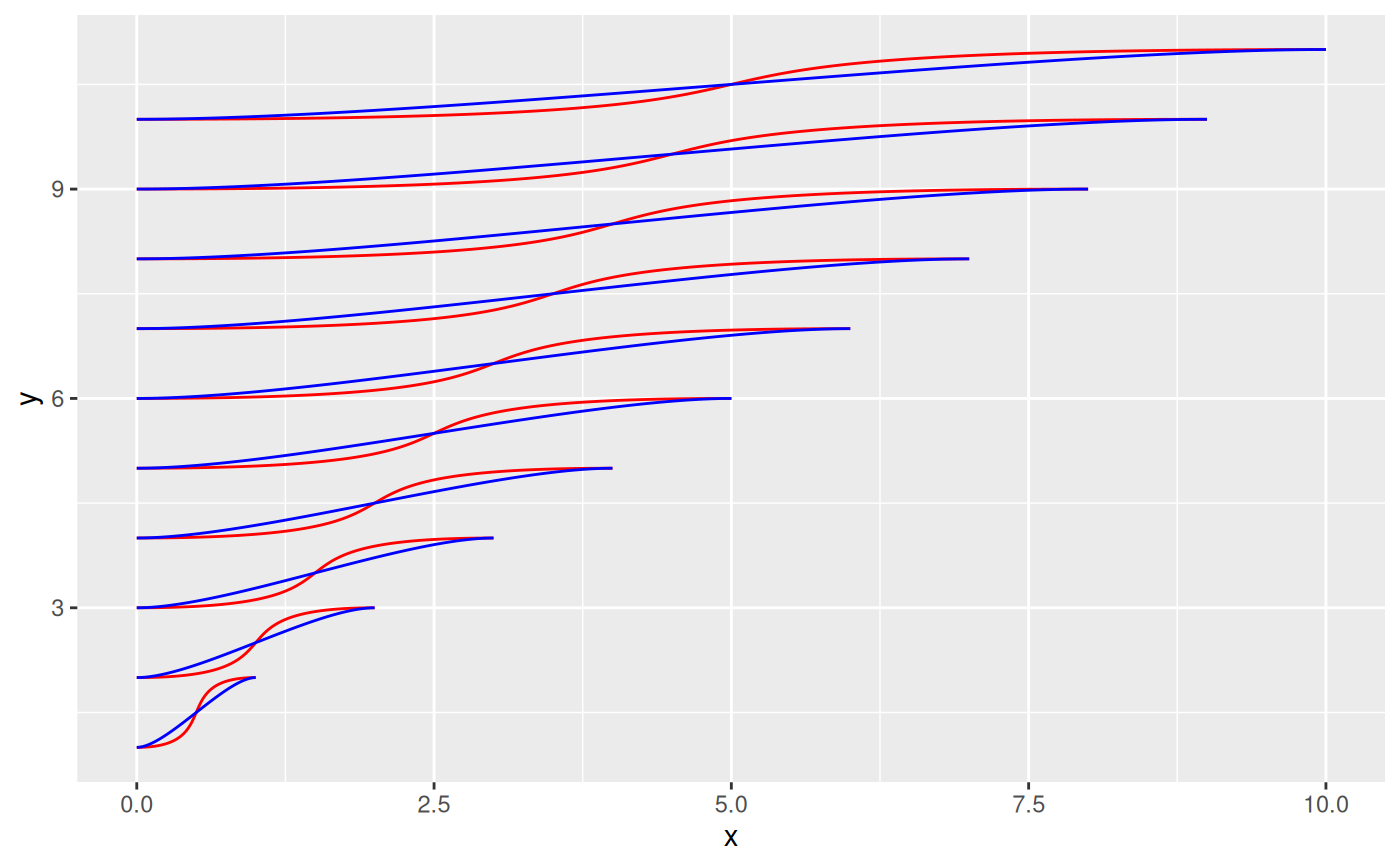A diagonal is a bezier curve where the control points are moved perpendicularly towards the center in either the x or y direction a fixed amount. The versions provided here calculates horizontal diagonals meaning that the x coordinate is moved to achieve the control point. The geom_diagonal() and stat_diagonal() functions are simply helpers that takes care of calculating the position of the control points and then forwards the actual bezier calculations to geom_bezier().

stat_diagonal(
mapping = NULL,
data = NULL,
geom = "path",
position = "identity",
n = 100,
strength = 0.5,
na.rm = FALSE,
orientation = NA,
show.legend = NA,
inherit.aes = TRUE,
...
)

geom_diagonal(
mapping = NULL,
data = NULL,
stat = "diagonal",
position = "identity",
n = 100,
na.rm = FALSE,
orientation = NA,
strength = 0.5,
show.legend = NA,
inherit.aes = TRUE,
...
)

stat_diagonal2(
mapping = NULL,
data = NULL,
geom = "path_interpolate",
position = "identity",
na.rm = FALSE,
orientation = NA,
show.legend = NA,
n = 100,
strength = 0.5,
inherit.aes = TRUE,
...
)

geom_diagonal2(
mapping = NULL,
data = NULL,
stat = "diagonal2",
position = "identity",
arrow = NULL,
lineend = "butt",
na.rm = FALSE,
orientation = NA,
show.legend = NA,
inherit.aes = TRUE,
n = 100,
strength = 0.5,
...
)

stat_diagonal0(
mapping = NULL,
data = NULL,
geom = "bezier0",
position = "identity",
na.rm = FALSE,
orientation = NA,
show.legend = NA,
inherit.aes = TRUE,
strength = 0.5,
...
)

geom_diagonal0(
mapping = NULL,
data = NULL,
stat = "diagonal0",
position = "identity",
arrow = NULL,
lineend = "butt",
na.rm = FALSE,
orientation = NA,
show.legend = NA,
inherit.aes = TRUE,
strength = 0.5,
...
)

## Arguments

mapping

Set of aesthetic mappings created by aes(). If specified and inherit.aes = TRUE (the default), it is combined with the default mapping at the top level of the plot. You must supply mapping if there is no plot mapping.

data

The data to be displayed in this layer. There are three options:

If NULL, the default, the data is inherited from the plot data as specified in the call to ggplot().

A data.frame, or other object, will override the plot data. All objects will be fortified to produce a data frame. See fortify() for which variables will be created.

A function will be called with a single argument, the plot data. The return value must be a data.frame, and will be used as the layer data. A function can be created from a formula (e.g. ~ head(.x, 10)).

geom

The geometric object to use to display the data, either as a ggproto Geom subclass or as a string naming the geom stripped of the geom_ prefix (e.g. "point" rather than "geom_point")

position

Position adjustment, either as a string naming the adjustment (e.g. "jitter" to use position_jitter), or the result of a call to a position adjustment function. Use the latter if you need to change the settings of the adjustment.

n

The number of points to create for each segment

strength

The proportion to move the control point along the x-axis towards the other end of the bezier curve

na.rm

If FALSE, the default, missing values are removed with a warning. If TRUE, missing values are silently removed.

orientation

The orientation of the layer. The default (NA) automatically determines the orientation from the aesthetic mapping. In the rare event that this fails it can be given explicitly by setting orientation to either "x" or "y". See the Orientation section for more detail.

show.legend

logical. Should this layer be included in the legends? NA, the default, includes if any aesthetics are mapped. FALSE never includes, and TRUE always includes. It can also be a named logical vector to finely select the aesthetics to display.

inherit.aes

If FALSE, overrides the default aesthetics, rather than combining with them. This is most useful for helper functions that define both data and aesthetics and shouldn't inherit behaviour from the default plot specification, e.g. borders().

...

Other arguments passed on to layer(). These are often aesthetics, used to set an aesthetic to a fixed value, like colour = "red" or size = 3. They may also be parameters to the paired geom/stat.

stat

The statistical transformation to use on the data for this layer, either as a ggproto Geom subclass or as a string naming the stat stripped of the stat_ prefix (e.g. "count" rather than "stat_count")

arrow

Arrow specification, as created by grid::arrow().

lineend

Line end style (round, butt, square).

## Aesthetics

geom_diagonal and geom_diagonal0 understand the following aesthetics (required aesthetics are in bold):

• x

• y

• xend

• yend

• color

• linewidth

• linetype

• alpha

• lineend

geom_diagonal2 understand the following aesthetics (required aesthetics are in bold):

• x

• y

• group

• color

• linewidth

• linetype

• alpha

• lineend

## Computed variables

x, y

The interpolated point coordinates

index

The progression along the interpolation mapped between 0 and 1

## Orientation

This geom treats each axis differently and, thus, can thus have two orientations. Often the orientation is easy to deduce from a combination of the given mappings and the types of positional scales in use. Thus, ggplot2 will by default try to guess which orientation the layer should have. Under rare circumstances, the orientation is ambiguous and guessing may fail. In that case the orientation can be specified directly using the orientation parameter, which can be either "x" or "y". The value gives the axis that the geom should run along, "x" being the default orientation you would expect for the geom.

## Examples

data <- data.frame(
x = rep(0, 10),
y = 1:10,
xend = 1:10,
yend = 2:11
)

ggplot(data) +
geom_diagonal(aes(x, y, xend = xend, yend = yend))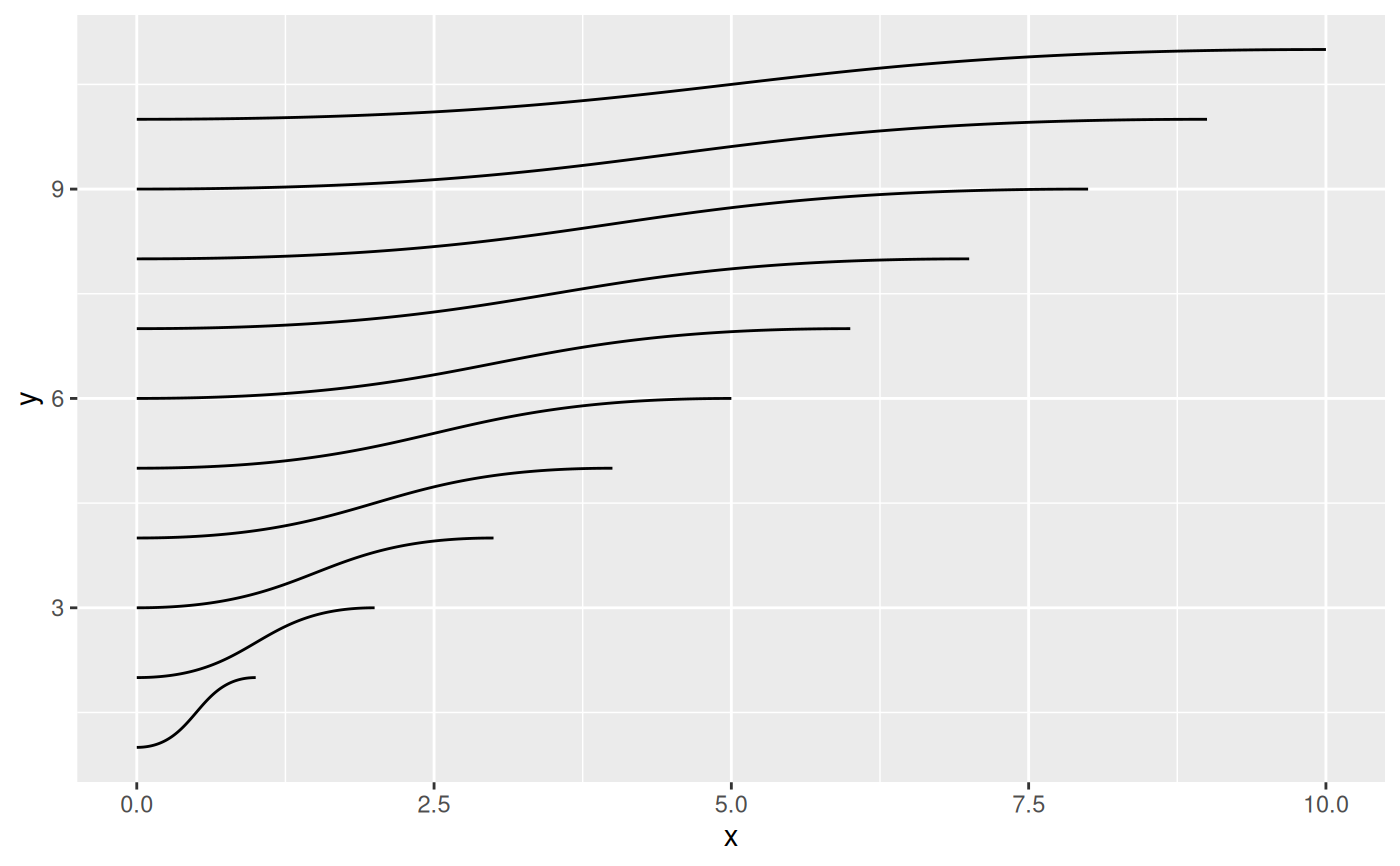# The standard version provides an index to create gradients
ggplot(data) +
geom_diagonal(aes(x, y, xend = xend, yend = yend, alpha = after_stat(index)))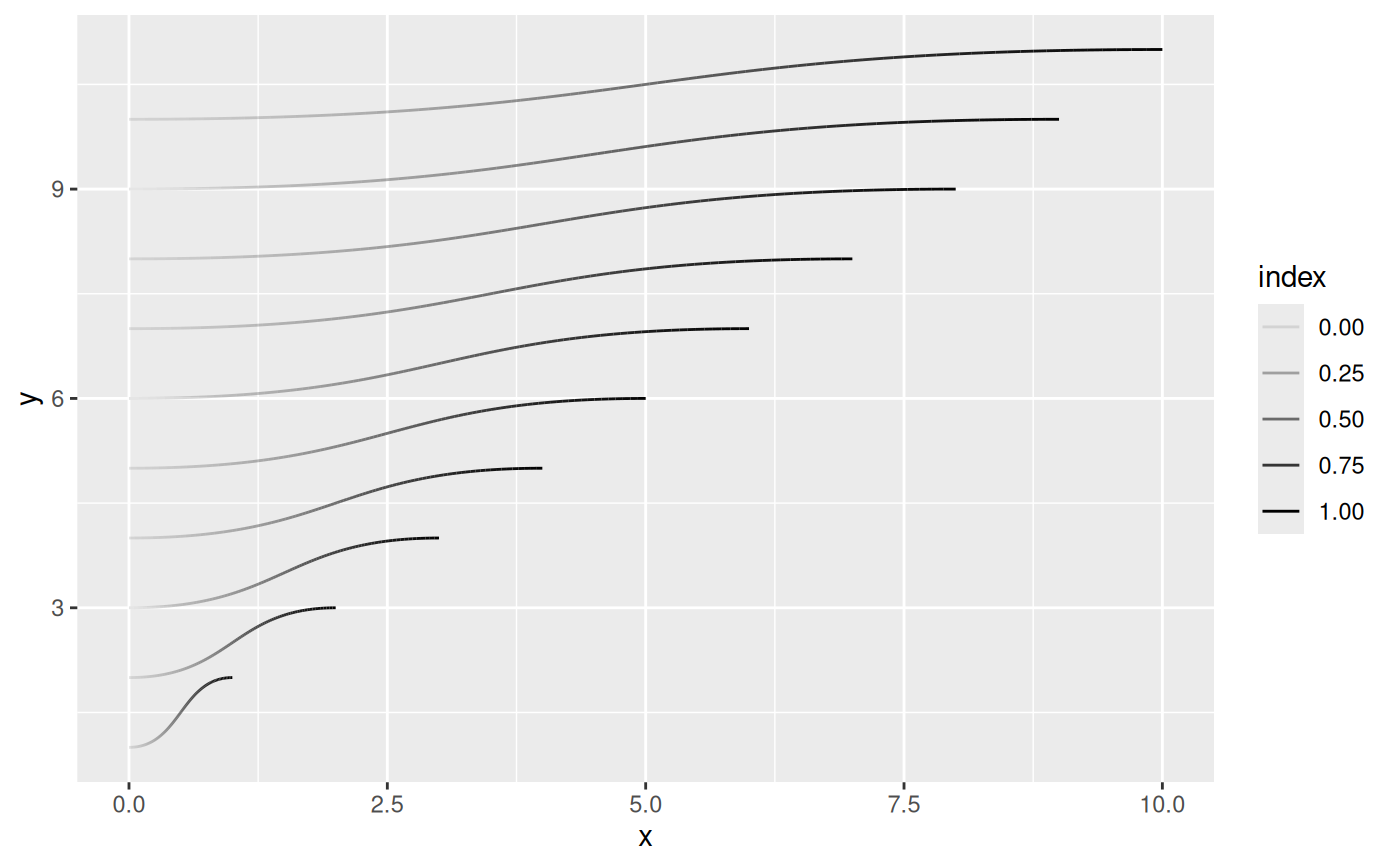# The 0 version uses bezierGrob under the hood for an approximation
ggplot(data) +
geom_diagonal0(aes(x, y, xend = xend, yend = yend))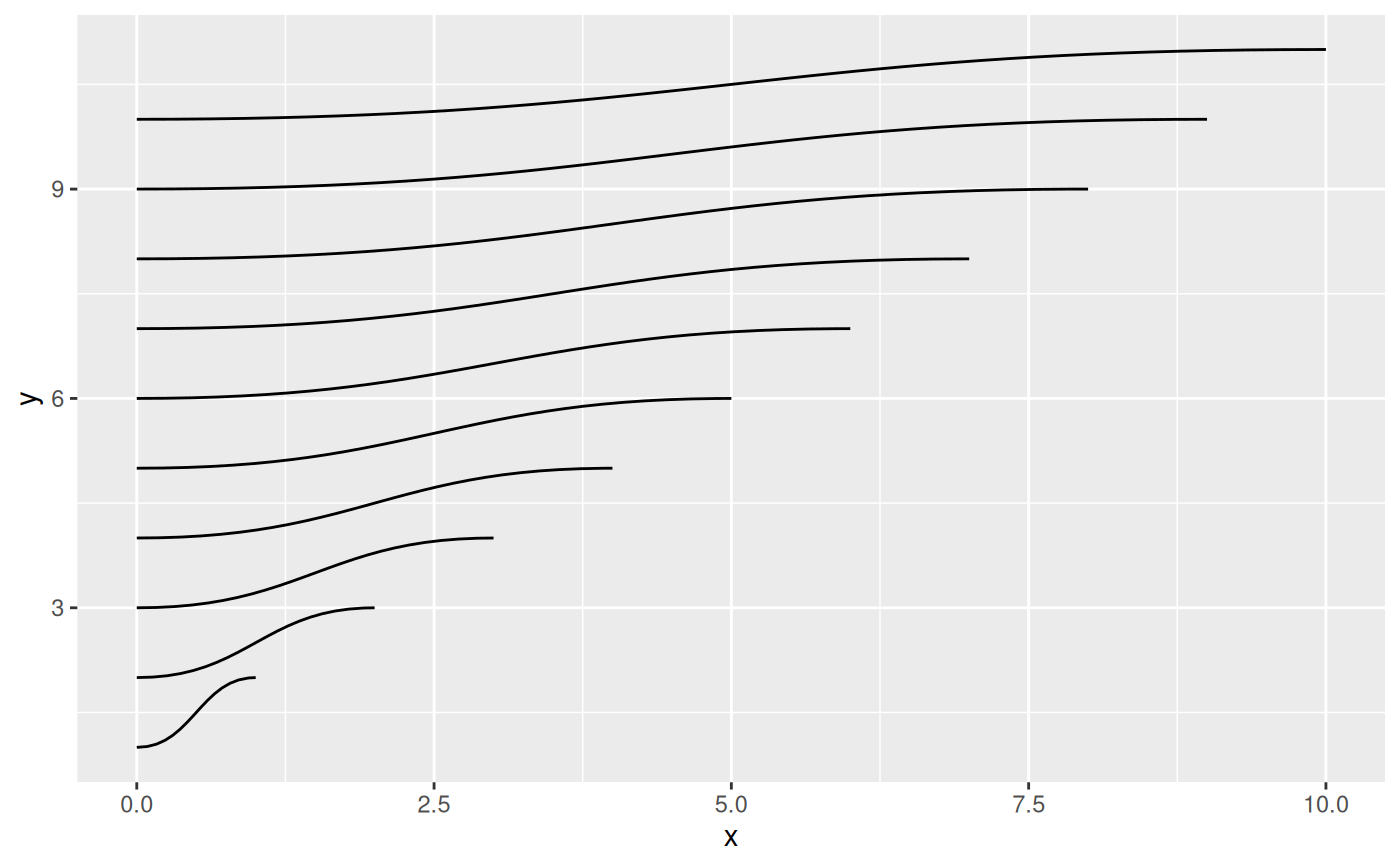# The 2 version allows you to interpolate between endpoint aesthetics
data2 <- data.frame(
x = c(data$x, data$xend),
y = c(data$y, data$yend),
group = rep(1:10, 2),
colour = sample(letters[1:5], 20, TRUE)
)
ggplot(data2) +
geom_diagonal2(aes(x, y, group = group, colour = colour))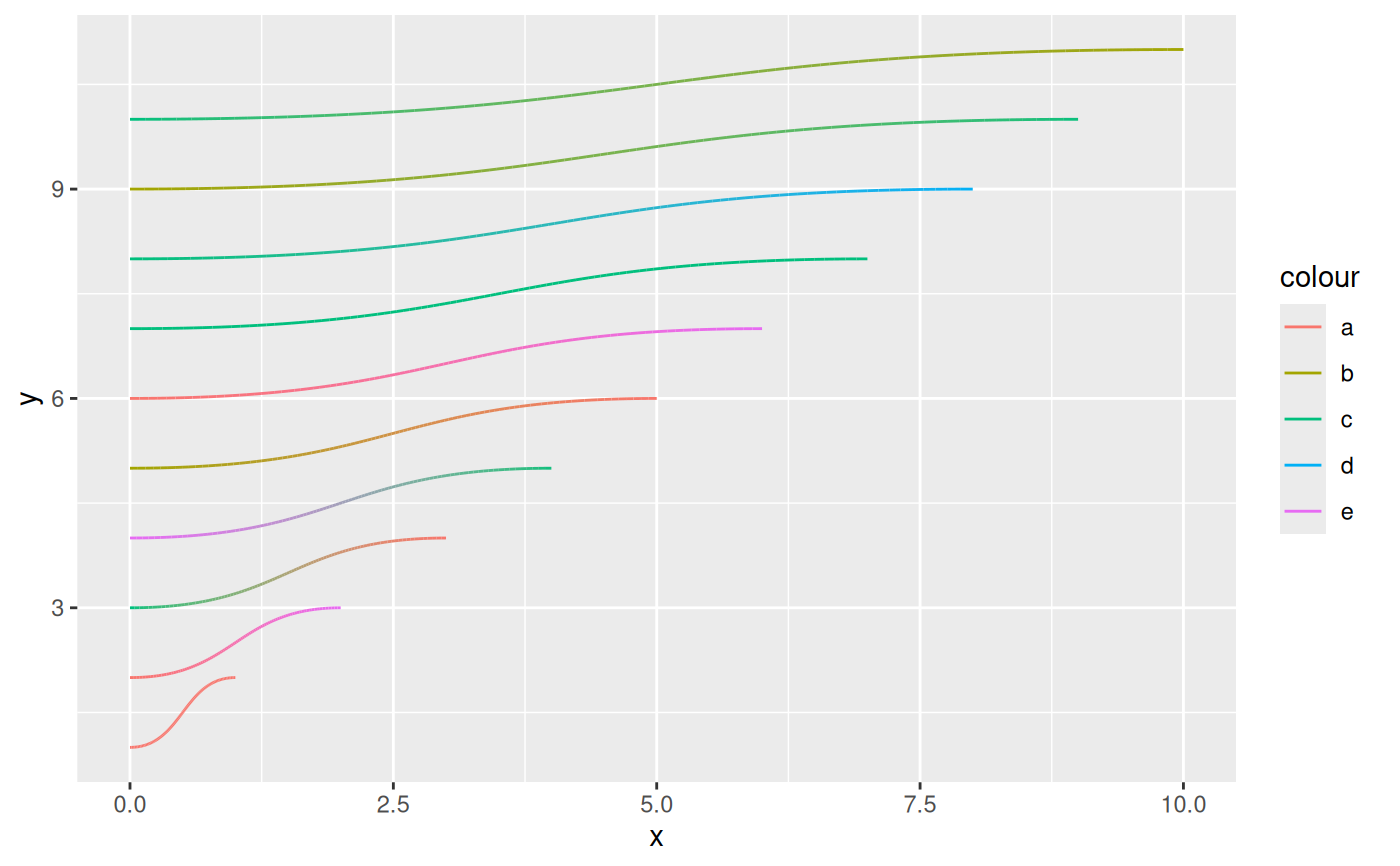# Use strength to control the steepness of the central region
ggplot(data, aes(x, y, xend = xend, yend = yend)) +
geom_diagonal(strength = 0.75, colour = 'red') +
geom_diagonal(strength = 0.25, colour = 'blue')